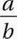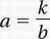# SAT Math Multiple Choice Question 771: Answer and Explanation

### Test Information

Question: 771

6. If the variable a varies inversely as b, which of the following statements must be true?

• A. a + b is a constant.
• B. a - b is a constant.
• C.is a constant.
• D. ab is a constant.

Recall from Chapter 8, Lesson 4, that if a is inversely proportional to b, then, where k is some positive constant. If we multiply both sides of this equation by b, we get ab = k, which means that the product ab is a constant.